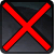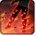# Missile BarrageConditions

## Related effects, buffs and debuffs

• [3s]Fire Zone
Taking elemental damage from standing in fire.

 Slot: Debuff Duration: 3s Tick rate: does not tick # occurrences: 1 Tags: tag.​abl.​flashpoint.​copero.​boss_bonus.​burning, tag.​abl.​debuff.​damage_over_time, tag.​abl.​dispel_type.​physical, tag.​abl.​flashpoint.​copero.​boss__bonus.​magma_pool Conditions: If target is an enemy
• On Apply

Perform the following actions:

• Play appearance epp . operation . iokath . trash . magma_droid . magma_burst_target_burn, dependent on calling effect
• On Apply

Only when the following conditions are met:

• If difficulty is set to 16-man

Perform the following actions:

• Spell Damage
- Unknown (609) = (bool) false
- Damage Type = (int) 3
- Slot = (int) 1
- Spell Type = (int) 2
- Level Cap = (int) 0
- Amount Max = (float) 0
- Amount Min = (float) 0
- Amount Percent = (float) 0
- Threat Percent = (float) 0
- Standard Health Percent Max = (float) 0.02
- Standard Health Percent Min = (float) 0.02
- Amount Modifier Percent = (float) 0
- Coefficient = (float) 0
- Health Steal Percentage = (float) 0
• On Apply

Only when the following conditions are met:

• If difficulty is set to 8-man SM

Perform the following actions:

• Spell Damage
- Unknown (609) = (bool) false
- Damage Type = (int) 3
- Slot = (int) 1
- Spell Type = (int) 2
- Level Cap = (int) 0
- Amount Max = (float) 0
- Amount Min = (float) 0
- Amount Percent = (float) 0
- Threat Percent = (float) 0
- Standard Health Percent Max = (float) 0.02
- Standard Health Percent Min = (float) 0.02
- Amount Modifier Percent = (float) 0
- Coefficient = (float) 0
- Health Steal Percentage = (float) 0
• On Apply

Only when the following conditions are met:

• If difficulty is set to 8-man HM

Perform the following actions:

• Spell Damage
- Unknown (609) = (bool) false
- Damage Type = (int) 3
- Slot = (int) 1
- Spell Type = (int) 2
- Level Cap = (int) 0
- Amount Max = (float) 0
- Amount Min = (float) 0
- Amount Percent = (float) 0
- Threat Percent = (float) 0
- Standard Health Percent Max = (float) 0.02
- Standard Health Percent Min = (float) 0.02
- Amount Modifier Percent = (float) 0
- Coefficient = (float) 0
- Health Steal Percentage = (float) 0